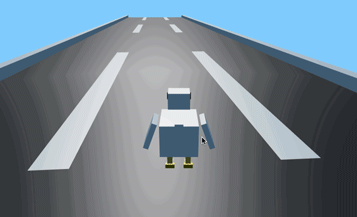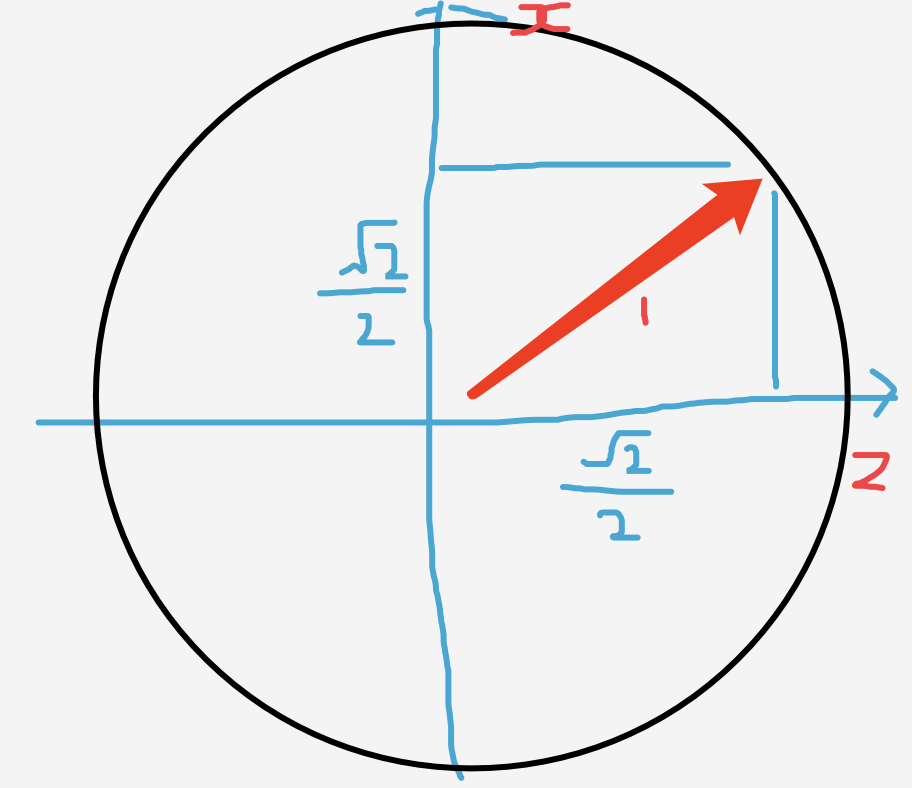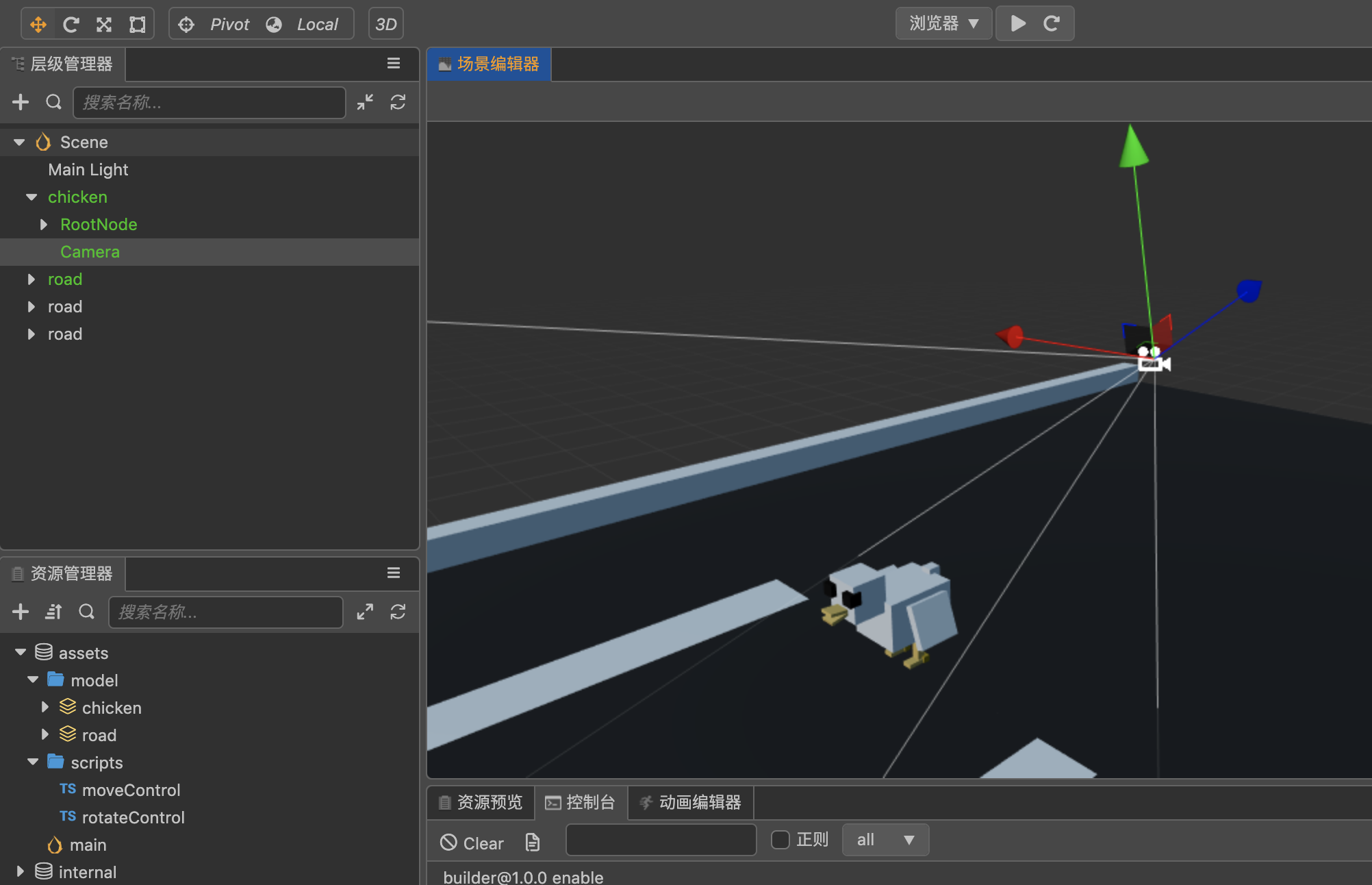# CocosCreator3D之视角移动

### 正文

#### 版本说明#### 模型的移动

let pos = this.node.getPosition();
pos.x += 10;
this.node.setPosition(pos);


// 根据自身方向，转化方向
_getDirection (x: number, y: number, z: number) {
const result = new Vec3(x, y, z);
math.Vec3.transformQuat(result, result, this.node.getRotation());
return result;
}const position = this.node.getPosition();
math.Vec3.scaleAndAdd(position, position, this._getDirection(1,0,0), deltaTime * this.speed);
this.node.setPosition(position);


#### 视角的旋转onMouseMove (event: EventMouse) {
const PI = 3.1415926535;
if(event.movementX != 0) {
const rotationY = this.node.getRotation();
math.Quat.rotateAround(rotationY, rotationY, this._getDirection(0, -1, 0), event.movementX / 5 / 360.0 * PI);
this.node.setRotation(rotationY);
}
if(event.movementY != 0) {
const rotationX = this.camera.getRotation();
math.Quat.rotateAround(rotationX, rotationX, this._getDirection(1, 0, 0), event.movementY / 5 / 360.0 * PI);
this.camera.setRotation(rotationX);
}
}


O(∩_∩)O~~

### 微信公众号©️2019 CSDN 皮肤主题: 技术工厂 设计师: CSDN官方博客